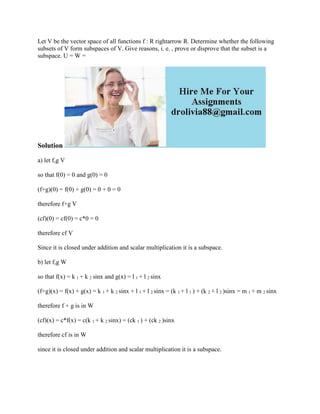Successfully reported this slideshow.

# Let V be the vector space of all functions f - R rightarrow R- Determi.docx×

# Let V be the vector space of all functions f - R rightarrow R- Determi.docx

Let V be the vector space of all functions f : R rightarrow R. Determine whether the following subsets of V form subspaces of V. Give reasons, i. e. , prove or disprove that the subset is a subspace. U = W =
Solution
a) let f,g V
so that f(0) = 0 and g(0) = 0
(f+g)(0) = f(0) + g(0) = 0 + 0 = 0
therefore f+g V
(cf)(0) = cf(0) = c*0 = 0
therefore cf V
Since it is closed under addition and scalar multiplication it is a subspace.
b) let f,g W
so that f(x) = k 1 + k 2 sinx and g(x) = l 1 + l 2 sinx
(f+g)(x) = f(x) + g(x) = k 1 + k 2 sinx + l 1 + l 2 sinx = (k 1 + l 1 ) + (k 2 + l 2 )sinx = m 1 + m 2 sinx
therefore f + g is in W
(cf)(x) = c*f(x) = c(k 1 + k 2 sinx) = (ck 1 ) + (ck 2 )sinx
therefore cf is in W
since it is closed under addition and scalar multiplication it is a subspace.
.

Let V be the vector space of all functions f : R rightarrow R. Determine whether the following subsets of V form subspaces of V. Give reasons, i. e. , prove or disprove that the subset is a subspace. U = W =
Solution
a) let f,g V
so that f(0) = 0 and g(0) = 0
(f+g)(0) = f(0) + g(0) = 0 + 0 = 0
therefore f+g V
(cf)(0) = cf(0) = c*0 = 0
therefore cf V
Since it is closed under addition and scalar multiplication it is a subspace.
b) let f,g W
so that f(x) = k 1 + k 2 sinx and g(x) = l 1 + l 2 sinx
(f+g)(x) = f(x) + g(x) = k 1 + k 2 sinx + l 1 + l 2 sinx = (k 1 + l 1 ) + (k 2 + l 2 )sinx = m 1 + m 2 sinx
therefore f + g is in W
(cf)(x) = c*f(x) = c(k 1 + k 2 sinx) = (ck 1 ) + (ck 2 )sinx
therefore cf is in W
since it is closed under addition and scalar multiplication it is a subspace.
.